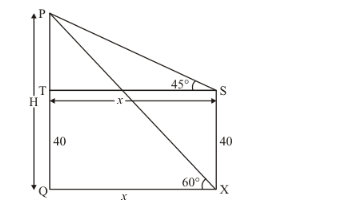# The angle of elevation of the top of a vertical tower PQ from a point X

Question:

The angle of elevation of the top of a vertical tower PQ from a point on the ground is 60°. At a point Y, 40 m vertically above X, the angle of elevation of the top is 45°. Calculate the height of the tower.

Solution:

Let PQ be the tower of height m and an angle of elevation of the top of tower PQ from point X is 60°. Angle of elevation at 40 m vertical from point X is 45°.

Let PQ = H m and SX = 40m. OX = x,.

Here we have to find height of tower.

The corresponding figure is as followsWe use trigonometric ratios.

In$\Rightarrow \quad \tan 45^{\circ}=\frac{h}{x}$

$\Rightarrow \quad 1=\frac{h}{x}$

$\Rightarrow \quad x=h$

Again in,

$\Rightarrow \quad \tan 60^{\circ}=\frac{h+40}{x}$

$\Rightarrow \quad \sqrt{3}=\frac{h+40}{x}$

$\Rightarrow \quad h+40=\sqrt{3} h$

$\Rightarrow h(\sqrt{3}-1)=40$

$\Rightarrow \quad h=\frac{40}{\sqrt{3}-1}$

$\Rightarrow \quad h=54.64$

Therefore $H=54.64+40$

$\Rightarrow H=94.64$

Hence the height of tower is $94.64 \mathrm{~m}$